## Material Balance for a Water Drive Mechanism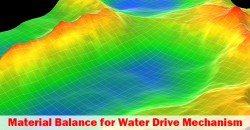This article will demonstrate a material balance equation in a natural water drive mechanism.  A full material balance equation is shown below: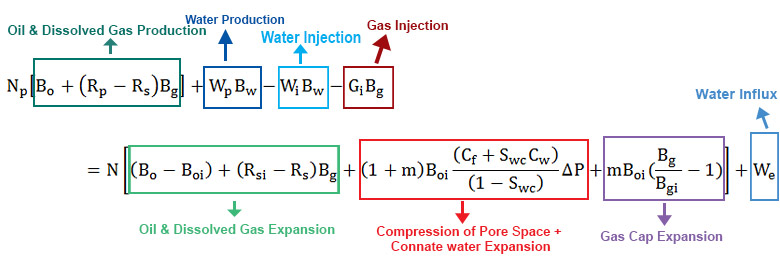Figure 1 – Full Material Balance Equation

Assumptions

• Reservoir pressure above the bubble point (Pb). Above a bubble point, Rs = Rsi.
• No water injection
• No gas injection
• No gas cap
• Neglect formation and connate water compressibility (Cf and Cw have little effect for a gas cap drive mechanism.)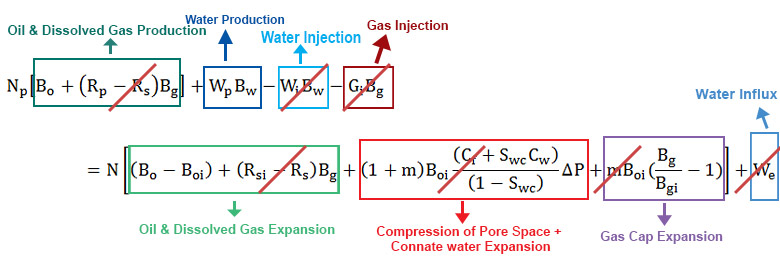Figure 2 – Material Balance Equation with Assumption for a Natural Water Drive Mechanism

## Material Balance for Gas Cap Drive Mechanism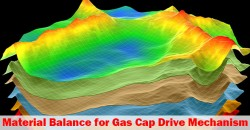Figure 1 – Full Material Balance Equation

Assumptions

• No water production
• No water injection
• No gas injection
• No water influx
• Neglect formation and connate water compressibility (Cf and Cw have little effect for a gas cap drive mechanism.)

## Solution Gas Drive Mechanism Explained in Material Balance Equation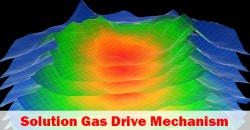Material balance equation can be applied for any drive mechanism and this article demonstrates how to apply the material balance equation in a solution drive mechanism. For a solution drive mechanism, there are 2 cases. The first case is when reservoir pressure is above a bubble point and the second case is when reservoir pressure is below a bubble point.

Solution Gas above Bubble PointFigure 1 – Full Material Balance Equation

## Material Balance Equation in Reservoir EngineeringMaterial balance is a mathematic way to express mass conservation in a reservoir and a simple key principle is “what reservoir is produced must be replaced by other mass.

Volume Produced = Volume Replaced

Volume Produced comes from Gas Production, Oil Production, and Water Production.

Volume Replaced comes from volume expansion, water in flux and water/gas injection.

Figure 1 shows the relationship of the material balance.Figure 1 – Concept of Material Balance

Let’s take a look at each component of equation. Continue reading